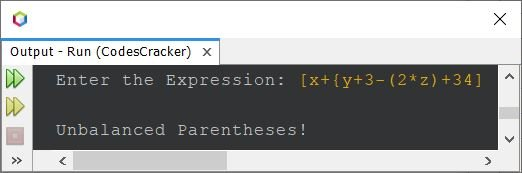# Java Program to Check Balanced Parentheses

This article contains a program in Java to check whether the expression has balanced parentheses or not. That is, the program helps in checking whether brackets used in an expression such as (, ), {, }, [, ] are in balance or not.

The expression will be in balanced parentheses, if there is an opening (, {, or [, then there must be a closing ), }, or ]. The program is created with and without using stack.

## Check Balanced Parentheses without using Stack

The question is, write a Java program to check balanced parentheses. The program must be created without using stack. And the string must be received by user at run-time. The program given below is the answer to this question:

```import java.util.Scanner;

public class CodesCracker
{
public static void main(String[] args)
{
String expression;
int i, length, j=0, count=0;
char ch, c;
char[] stk = new char;
Scanner s = new Scanner(System.in);

System.out.print("Enter the Expression: ");
expression = s.nextLine();

length = expression.length();

for(i=0; i<length; i++)
{
ch = expression.charAt(i);
if(ch=='(' || ch=='{' || ch=='[')
{
stk[j] = ch;
j++;
count = 1;
}
else if(ch==')')
{
if(count==1)
j--;
c = stk[j];
if(stk.length==0 || c != '(')
{
System.out.println("\nUnbalanced Parentheses!");
return;
}
}
else if(ch=='}')
{
if(count==1)
j--;
c = stk[j];
if(stk.length==0 || c != '{')
{
System.out.println("\nUnbalanced Parentheses!");
return;
}
}
else if(ch==']')
{
if(count==1)
j--;
c = stk[j];
if(stk.length==0 || c != '[')
{
System.out.println("\nUnbalanced Parentheses!");
return;
}
}
}
System.out.println("\nBalanced Parentheses.");
}
}```

Here is its sample run with user input {2+(4*2)-(3+5)}:Here is another sample run with user input [x+{y+3-(2*z)+34] as expression to check whether parentheses are balanced or not:## Check Balanced Parentheses using Stack

The program given below does the same job as of previous program. The only difference is its approach, as this program is created using stack.

```import java.util.*;

public class CodesCracker
{
public static void main(String[] args)
{
String expression;
int i, length;
char ch;
Scanner s = new Scanner(System.in);

System.out.print("Enter the Expression: ");
expression = s.nextLine();

Stack<Character> stack = new Stack<Character>();
length = expression.length();

for(i=0; i<length; i++)
{
ch = expression.charAt(i);
if(ch=='(' || ch=='{' || ch=='[')
{
stack.push(ch);
}
else if(ch==')')
{
if(stack.isEmpty() || stack.pop() != '(')
{
System.out.println("\nUnbalanced Parentheses!");
return;
}
}
else if(ch=='}')
{
if(stack.isEmpty() || stack.pop() != '{')
{
System.out.println("\nUnbalanced Parentheses!");
return;
}
}
else if(ch==']')
{
if(stack.isEmpty() || stack.pop() != '[')
{
System.out.println("\nUnbalanced Parentheses!");
return;
}
}
}
if(stack.isEmpty())
System.out.println("\nBalanced Parentheses.");
}
}```

This program produces same output as of previous program. In above program, the code:

`stack.isEmpty()`

is used to check whether the stack is empty or not. In previous program, I've done the same using following code:

`stk.length==0`

where stk is character array, used to store parentheses.

The code stack.push() is used to push or insert an element at top of the stack, whereas the code stack.pop() is used to remove the top element from the stack.

Java Online Test

« Previous Program Next Program »